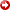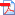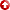--> --> Seismic Rock-Property Transforms for Estimating Lithology and Pore-Fluid Content, by Haitao Ren, Fred J. Hilterman, Zhengyun Zhou, and Mritunjay Kumar, #40224 (2006).
[First Hit]#Click to view article in PDF format.

Seismic Rock-Property Transforms for Estimating Lithology and Pore-Fluid Content*

By

Haitao Ren1, Fred J. Hilterman1, Zhengyun Zhou1, and Mritunjay Kumar2,

Search and Discovery Article #40224

Posted November 30, 2006

*Adapted from extended abstract prepared for presentation at AAPG Annual Convention, Houston, Texas, April 9-12, 2006

1Center for Applied Geosciences and Energy, University of Houston ([email protected])

2Dept. of Geosciences, University of Houston

Velocity and density values from approximately 2200 sand reservoirs and their encasing shale intervals were cataloged using well-log curves from the offshore Louisiana shelf in the Gulf of Mexico. The reservoir depths range from 200m to 5500m and the reservoirs are predominantly Pliocene to mid-Miocene in age. Fluid substitution was conducted so that all 2200 reservoirs have velocity and density values for gas, oil and brine saturation. While conventional depth plots for velocity and density trends were unstable and generally exhibited random correlation, we did discover two robust reflection-coefficient transforms. The first transform relates the normal-incidentreflection coefficient (NI) for either gas or oil saturation to the NI of the equivalent brine-saturated reservoir. We call these pore-fluid transforms. The second transform relates the near-angle reflection amplitude to the far-angle reflection amplitude for various pore-fluid saturations. Surprisingly, the change in amplitude from near to far angles is predominantly dependent on lithology (shale content, porosity, etc.) and not the pore-fluid saturant. Thus, these relationships are named lithology transforms. Using a lithology transform along with the horizon amplitude maps from near- and far-angle stacks, a reflection-coefficient map for a specific pore fluid is generated. Normally, the first reflection coefficient map generated is for the down-dip brine-saturated portion of the prospect, and then it is changed to represent the reflection coefficient values for various hydrocarbon saturations using the pore-fluid transforms. When the converted reflection coefficient values of the down-dip portion of the prospect match the prospect reflection coefficient values, then an estimate of the pore fluid and water saturation, SW, is established.

 uSummary uFigure captions uIntroduction uCalibration uMapping uExample uDiscussion uConclusions uAcknowledgements uReferences                                                   uSummary uFigure captions uIntroduction uCalibration uMapping uExample uDiscussion uConclusions uAcknowledgements uReferences                                                 uSummary uFigure captions uIntroduction uCalibration uMapping uExample uDiscussion uConclusions uAcknowledgements uReferences                                                   uSummary uFigure captions uIntroduction uCalibration uMapping uExample uDiscussion uConclusions uAcknowledgements uReferences                                                   uSummary uFigure captions uIntroduction uCalibration uMapping uExample uDiscussion uConclusions uAcknowledgements uReferences                                                   uSummary uFigure captions uIntroduction uCalibration uMapping uExample uDiscussion uConclusions uAcknowledgements uReferences Distinguishing between fizz and commercial gas saturations from seismic data is a difficult problem because low and high gas saturation result in very similar Amplitude-Versus-Offset (AVO) responses. A small amount of gas in the pore fluid lowers the rock P-wave velocity (Vp) dramatically, while the S-wave velocity (Vs) does not change much for different pore fluids and saturations. Rock bulk density changes linearly with water-saturation. Consequently, fizz water and commercial gas saturations have very similar Vp and Vp/Vs values and subsequently very similar AVO responses. Hilterman and Liang (2004) showed that a reservoir with 31% porosity and SW=0.3 has an identical AVO response to a reservoir with 34% porosity and SW=0.9. In short, an AVO analysis of the prospect reservoir, by itself, will not discriminate economic versus fizz saturation. However, by comparing the reservoir’s AVO properties to the down-dip water-saturated AVO response, Zhou et al. (2005) were able to distinguish fizz from economic gas saturation. In this paper, we extend the work presented by Zhou et al. by presenting two robust transforms developed from a large rock-property database and demonstrate the technique with seismic field data.   Rock-properties for approximately 2200 60m-thick intervals were available from the proprietary TILETM 2 database in the Gulf of Mexico (Geophysical Development Corporation (GDC), Figure 1). For each interval, the velocity and density for shale and for wet-, gas- and fizz-saturated sands were calculated using fluid-substitution techniques (Hilterman, 2001). With the velocity and density values known or estimated, NI values were calculated. Finally, using the linear approximation ofZoeppritz’s equation, the reflection coefficients for wet, gas, and fizz saturations at anincidentangle of 30° were calculated for the top of each reservoir. From the catalog of reflection-coefficients, pore-fluid and lithology transforms were developed for the different pore-fluid states and these are shown in Figure 2. In Figure 2a, the NI for both gas and fizz saturation are plotted against the NI of the equivalent brine-saturated reservoir. These are a pore-fluid transforms. In Figure 2b, NI is plotted against the far-angle reflection coefficient. There is a separate plot for each of the pore-fluids. Surprisingly, the change in amplitude from near to far offsets is predominantly dependent on lithology (shale content, porosity, etc) and not the pore-fluid saturant. Thus, this type is named the lithology transform. The quantitative relationships for pore-fluid and lithology transforms are expressed by NIHYC = B1 + B2 NIWET                                            (1a) RC(θ) = L1 + L2 NI                                                    (1b) where θ is the reflectionincidentangle; RC(θ) is the reflection coefficient at the far-offset angle θ (set to 30° for this study); NIHYC and NIWET relate to the hydrocarbon and brine saturations respectively. B1, B2, L1and L2 are the transform coefficients and are listed in Table 1.   Zhou et al. (2005) proposed a technique for converting seismic amplitude maps into NI maps. The technique is based on a thin-bed reflection model that is expressed by Lin and Phair (1993) as A(θ ) = K *RC(θ )*cos(θ ) ,                                         (2) Where θ is theincidentangle; A(θ) is the seismic amplitude; RC(θ) is reflection coefficient for the upper boundary. K is expressed by, K = k * 4πb/λ , Where k is a constant for the seismic survey; b is the thin-bed thickness; and, λ is the seismic wavelength. The lithology transform that yields NI is NI = (L1*A0°) / [A(θ)/cos(θ) – L2*A(0°)] .                 (3) Before converting the seismic near- and far-angle stacks, A(0°) and A(θ=30°), into a reflection coefficient map, a preprocessing multiplication is necessary. Normally, the amplitudes on the far-angle stack are not properly calibrated to the near-angle stack. This initial calibration of the angle stacks to borehole data is very important in the NI mapping technique.   Assume the estimated normal incidence, NIest, from the field data near- and far-angle stacks is P times higher than the true normal incidence, NItrue. That is, NIest=P*NItrue. Then, a new far-angle stack, Atrue(θ) can be generated from the original angle stacks Araw(θ) and Araw(0°). It is reasonable to assume that the amplitudes for Araw(0°) are valid and only the amplitudes for Araw(θ) need to be corrected. With a little bit of algebra, the following results. Atrue(θ) = P*Araw(θ) – cos(θ) *L1* Araw(0°)*(P-1), and           (4a) Atrue(θ) = Araw(0°)                                                                     (4b) The calibrated amplitude maps fromEquations4a and 4b are now inserted into Equation     Figure 3 contains horizon amplitude plots from an offshore block (3 miles by 3 miles) in High Island, Gulf of Mexico. Figure 3a is the near-angle map and Figure 3b, the far-angle. The area circled by a red line, which we will call a prospect, is actually a known commercial gas field. Using a suite of well-log curves from a field well, the NItrue values for wet, fizz and gas saturation were generated for the location of the well. Equation 3 was applied to the two angle stack maps with the wet coefficients in Table 1 to obtain a reflection-coefficient map that pertains to the areas where the sand formations were wet. The amplitudes down-dip from the gas field (which should be brine saturated) were then analyzed to obtain the normalincidentvalue, NIest. The down-dip brine-saturation value [NIest] was slightly different from the NItrue predicted from well-log fluid substitution. The ratio of NIest/ NItrue yields a value for P. A new far-angle stack was generated using Equation 4a. It is not essential that a field well be used for calibration. In fact, a well anywhere in the survey area that penetrated the horizon event could be used for calibration. With the amplitudes of the near- and far-angle stacks calibrated by a well, the water-saturation estimation technique of Zhou et al. was continued.   The first step is to convert all the brine-saturated formations into their respective NI map. This is done by applying Equation 3 with the wet coefficients from Table 1 to yield the NI wet map in Figure 4a. The prospect is masked because we believe it is not brine saturated and thus the computed NI values would be incorrect. Even though the lithology or bed thickness may change across the survey, this map should be a good estimate of the brine-saturated NI; that is, NI for the brine-saturated formations may vary. Using Equation 1 and the fizz coefficients in Table 1, the brine-saturated map in Figure 4a is converted to an equivalent fizz reflection coefficient map as shown by Figure 4d. In essence, a fluid substitution was performed on the wet NI map in Figure 4a. A similar process is done to obtain the gas NI map in Figure 4e.   We will set aside the maps in Figures 4d and 4e for a moment and return to the near- and far-angle maps in Figure 3. This time, we will assume that the prospect is fizz charged (SW=0.9) and apply Equation 3 to the two angle stack maps using the appropriate fizz coefficients in Table 1. The result is displayed in Figure 4b, where only the prospect area is open because we are applying a lithology transform that assumes fizz saturation. Figure 4c represents a similar lithology transform under the assumption that at the prospect SW= 0.3 (gas).   The final step is to compare the two prediction processes. If the prospect is truly fizz charged, then the amplitude in the prospect (Figure 4b) should match the NI down-dip from the prospect that was fluid substituted (Figure 4d).   In the combined fizz case map (Figure 4d), the color of prospect area was calculated by equation (3), and this color is significantly different from that of the outside area computed by Equation (1). This means that, in the prospect area, the assumption of a fizz reservoir is wrong. However, in the combined gas case map (Figure 4e), the color of prospect area matches the outside area quite well, which shows that the assumption that the prospect is a commercial gas reservoir is correct.   There are several advantages of this interpretation technique to estimate SW. First, there is no requirement that the down-dip wet zone has the same bed thickness as the prospect zone. Second, the knowledge of wavelet phase is not critical. Third, the lithology transform is locally derived, and it allows for changes in porosity, shale content, cementation, etc that normally effect an inversion result. Fourth, calibrating the far-angle stack to the near-angle stack to compensate for incorrect gain functions applied during seismic processing is an easy step.   There are also some limitations. This method assumes that the down-dip wet zone and the prospect area have the same rock type and similar porosity. It requires that the down-dip wet zone is available. The method might not be applicable in complicated structures where it is difficult to ascertain if a down-dip wet zone exists.   Normalincidentreflection coefficients vary with changing rock properties. But NIGAS and NIWET locally have a robust linear relationship that we called the pore-fluid transform. For specific pore-fluids and SW, far-angle reflection coefficients linearly relate to NI. This we named the lithology transform.   An estimation of SW was possible once the two rock-property transforms were developed. The technique requires an amplitude comparison of the area down-dip from the prospect in order to predict pore-fluid content. It is the difference in the normal incidence between the brine-saturated formation and the prospect that governs the prediction.   We thank the sponsors of the Reservoir Quantification Laboratory. We thank Fairfield for the seismic data and GDC for the rock-properties in TILE2. Portions of this work were prepared with the support of the U.S. Department of Energy under the Award No. DE-FC26-04NT15503. However, any opinions, findings, conclusions, or recommendations expressed herein are those of the authors and do not necessary reflect the views of DOE.   Hilterman, F.J., 2001, Seismic amplitude interpretation: Distinguished Instructor Series, No. 4, SEG/EAGE. Hilterman, F., and Liang, L,, 2003, Linking rock-property trends and AVOequationsto GOM deep-water reservoirs: 73rd Ann. Internat. Mtg, Soc. Expl. Geophys., p. 211-214. Lin. T.L., and Phair. R., 1993, AVO tuning: 63rd Ann. Internat. Mtg., Soc. Expl. Geophys., Expanded Abstracts, p. 727-730. Zhou, Z., Hilterman, F., Ren, H., and Kumar, M., 2005, Water-saturation estimation from seismic and rock-property trends: 75th Ann. Internat. Mtg: Soc. Expl. Geophys.